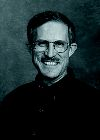Mathematics: The Statue in the Stone

Among his many other projects, Bailey has developed software that translates ordinary FORTRAN programs into programs capable of arbitrary precision—calculations accurate to tens, hundreds, or even many thousands of digits. Real physical problems that require this kind of "multiprecision" include, for example, simulating the dynamics of vortices, in which 80- to 100-digit arithmetic is needed for extremely high accuracy in each of many sequential calculations, to avoid meaningless results at the end.

Because the integer-relation detection algorithm known as PSLQ* is among the kinds of computation that need very high precision arithmetic to avoid nonsense results, "the new method wasn’t practical until it could be implemented with multiprecision software on high-performance computer systems," Bailey says.DAVID BAILEYDavid Bailey joined NERSC in 1998 after two decades of contributions to supercomputing at NASA, SRI International, and the Department of Defense, following a doctorate from Stanford and a B.S. from Brigham Young. The math life has brought Bailey a raft of prizes and honors, but what best characterizes his contributions, from pure theory to performance standards, is his sense of mathematical delight. Case in point, Bailey’s motto (with no apologies to Descartes): "computo ergo sum."

He calls PSLQ "a computer algorithm to discover new mathematical results." As a tool of experimental mathematics, PSLQ’s purpose is to discover new mathematical relations among numbers—for example, constants that occur in various mathematical formulas.

In a short time PSLQ has found polylogarithmic formulas in algebraic number theory, identified a class of multiple-sum constants, uncovered relations in the renormalization procedures of quantum field theory symbolized by Feynman diagrams, and found a formula for calculating individual digits of pi.

The last may be its most startling result: a simple formula for calculating any binary digit of pi without calculating the digits preceding it. Before PSLQ, mathematicians had not thought such a digit-extraction algorithm for pi was possible.

With the remarkably simple pi formula even a personal computer can calculate pi’s millionth binary digit in just a few seconds. (The formula works only for binary digits, however, not decimal digits.)

Some of PSLQ’s results have profound implications. The new pi formula suggests an avenue, which Bailey and mathematician Richard Crandall are now pursuing, for investigating the long-held but never proved assumption that pi’s digits are random. The Feynman-diagram results suggest unsuspected relationships among formulas associated with fundamental particles.

Helaman Ferguson is a noted sculptor as well as mathematician; one of his works, The Eight-Fold Way, stands in the courtyard of the Mathematical Sciences Research Institute on the hill above Berkeley Lab. Ferguson has described using Euclid’s algorithm to find the largest common divisor of two numbers as a process of discarding the excess until the divisor is revealed.

"It is also true that my sculptural form of expression is subtractive: I get my mathematical forms by direct carving of stone," says Ferguson, in terms highly reminiscent of "the statue in the stone," the Renaissance conceit that sculpture is merely a process of chipping away the excess marble to reveal the statue already inside.

For finding relationships between more than just two numbers, a sharper chisel is needed. With Bailey’s software implementing it, the PSLQ algorithm provides a new tool to reveal innate but previously hidden integer relations.

"In the case of my sculpture, the direct carving is an aesthetic choice," says Ferguson, noting that particularly for the PSLQ algorithm, "it seems more of a necessity. But then what isn’t an aesthetic choice in mathematics?"

Ferguson’s and Bailey’s description of a practical PSLQ algorithm first appeared in print in January, 1999. Just one year later Computing in Science and Engineering magazine, published by the Institute of Electrical and Electronics Engineers, named PSLQ one of the top 10 "Algorithms of the Century." The editors described PSLQ as "a centerpiece of the emerging discipline of ‘experimental mathematics’—the use of modern computer technology as an exploratory tool in mathematical research."

PSLQ, an efficient, numerically stable algorithm guaranteed to find relations among integers in a limited number of iterations, is a shining example of modern computer technology applied to the discovery of new facts of mathematics and physics—truly an algorithm of the century. It’s also a harbinger of the future: experimental mathematics using computers will become increasingly important in the coming century.

Bailey’s technical papers on the PSLQ algorithm and related discoveries can be downloaded from the web at http://www. nersc.gov/~dhbailey. A gallery of Ferguson’s sculpture is on view at http://www.helasculpt.com/.  — Paul Preuss

* from its use of a partial-sum-of-squares (PS) vector and lower-diagonal-orthogonal (LQ) matrix factorization

 < Highlights Top ^
Next >